Hostname: page-component-588bc86c8c-schgg Total loading time: 0 Render date: 2023-11-30T07:52:06.244Z Has data issue: false Feature Flags: { "corePageComponentGetUserInfoFromSharedSession": true, "coreDisableEcommerce": false, "useRatesEcommerce": true } hasContentIssue false

# A NOTE ON THE DIOPHANTINE EQUATION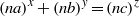$\mathop{(na)}\nolimits ^{x} + \mathop{(nb)}\nolimits ^{y} = \mathop{(nc)}\nolimits ^{z}$

Published online by Cambridge University Press:  07 August 2013

## Abstract

Core share and HTML view are not possible as this article does not have html content. However, as you have access to this content, a full PDF is available via the ‘Save PDF’ action button.

Let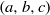$(a, b, c)$ be a primitive Pythagorean triple satisfying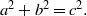${a}^{2} + {b}^{2} = {c}^{2} .$ In 1956, Jeśmanowicz conjectured that for any given positive integer$n$ the only solution of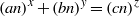$\mathop{(an)}\nolimits ^{x} + \mathop{(bn)}\nolimits ^{y} = \mathop{(cn)}\nolimits ^{z}$ in positive integers is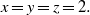$x= y= z= 2.$ In this paper, for the primitive Pythagorean triple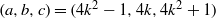$(a, b, c)= (4{k}^{2} - 1, 4k, 4{k}^{2} + 1)$ with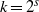$k= {2}^{s}$ for some positive integer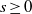$s\geq 0$, we prove the conjecture when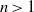$n\gt 1$ and certain divisibility conditions are satisfied.

Type
Research Article
Information

## References

Dem´janenko, V. A., ‘On Jeśmanowicz’ problem for Pythagorean numbers’, Izv. Vyss. Ucebn. Zaved. Mat. 48 (1965), 5256 (in Russian).Google Scholar
Deng, M. J., ‘On the Diophantine equation$\mathop{(15n)}\nolimits ^{x} + \mathop{(112n)}\nolimits ^{y} = \mathop{(113n)}\nolimits ^{z}$’, J. Natur. Sci. Heilongjiang University 24 (2007), 617620 (in Chinese).Google Scholar
Deng, M. and Cohen, G. L., ‘On the conjecture of Jeśmanowicz concerning Pythagorean triples’, Bull. Aust. Math. Soc. 57 (1998), 515524.Google Scholar
Jeśmanowicz, L., ‘Several remarks on Pythagorean numbers’, Wiad. Mat. 1 (1955–1956), 196202 (in Polish).Google Scholar
Le, M. H., ‘A note on Jeśmanowicz’ conjecture concerning Pythagorean triples’, Bull. Aust. Math. Soc. 59 (1999), 477480.Google Scholar
Lu, W. T., ‘On the Pythagorean numbers$4{n}^{2} - 1$;$4n$ and$4{n}^{2} + 1$’, Acta Sci. Natur. Univ. Szechuan 2 (1959), 3942 (in Chinese).Google Scholar
Miyazaki, T., ‘On the conjecture of Jeśmanowicz concerning Pythagorean triples’, Bull. Aust. Math. Soc. 80 (2009), 413422.Google Scholar
Miyazaki, T., ‘Generalizations of classical results on Jeśmanowicz’ conjecture concerning Pythagorean triples’, J. Number Theory 133 (2013), 583595.Google Scholar
Sierpiński, W., ‘On the equation${3}^{x} + {4}^{y} = {5}^{z}$’, Wiad. Mat. 1 (1955–1956), 194195 (in Polish).Google Scholar
Tang, M. and Yang, Z. J., ‘Jeśmanowicz’ conjecture revisited’, Bull. Aust. Math. Soc., to appear; available online, doi:10.1017/S0004972713000038.Google Scholar
Yang, Z. J. and Tang, M., ‘On the Diophantine equation$\mathop{(8n)}\nolimits ^{x} + \mathop{(15n)}\nolimits ^{y} = \mathop{(17n)}\nolimits ^{z}$’, Bull. Aust. Math. Soc. 86 (2012), 348352.Google Scholar